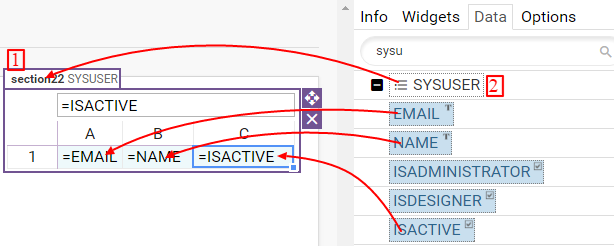Data option shows which Data object is bound to section.

Spreadsheet and Table can be bound to Data, that means that Spreadsheet section can be connected to one data record, and Table section can be connected to multiple data records.

Columns from Data object can be used in spreadsheet formulas.

There are two ways to bind Data object to Section:

• Drag data object (2) from Data tab to section name (1), then one can drag data columns to section cells, then edit lookup formula.
• Write lookup formula in Filter option.

Examples of lookup formulas:

=LOOKUP(SYSUSER) - Data SYSUSER, no query

=LOOKUP(PRODUCT, id=123) - Data PRODUCT, get product with ID 123

=LOOKUP(PRODUCT, price > 100) - Data PRODUCT, get products with price greater than 100Dragging Data object and columns on section